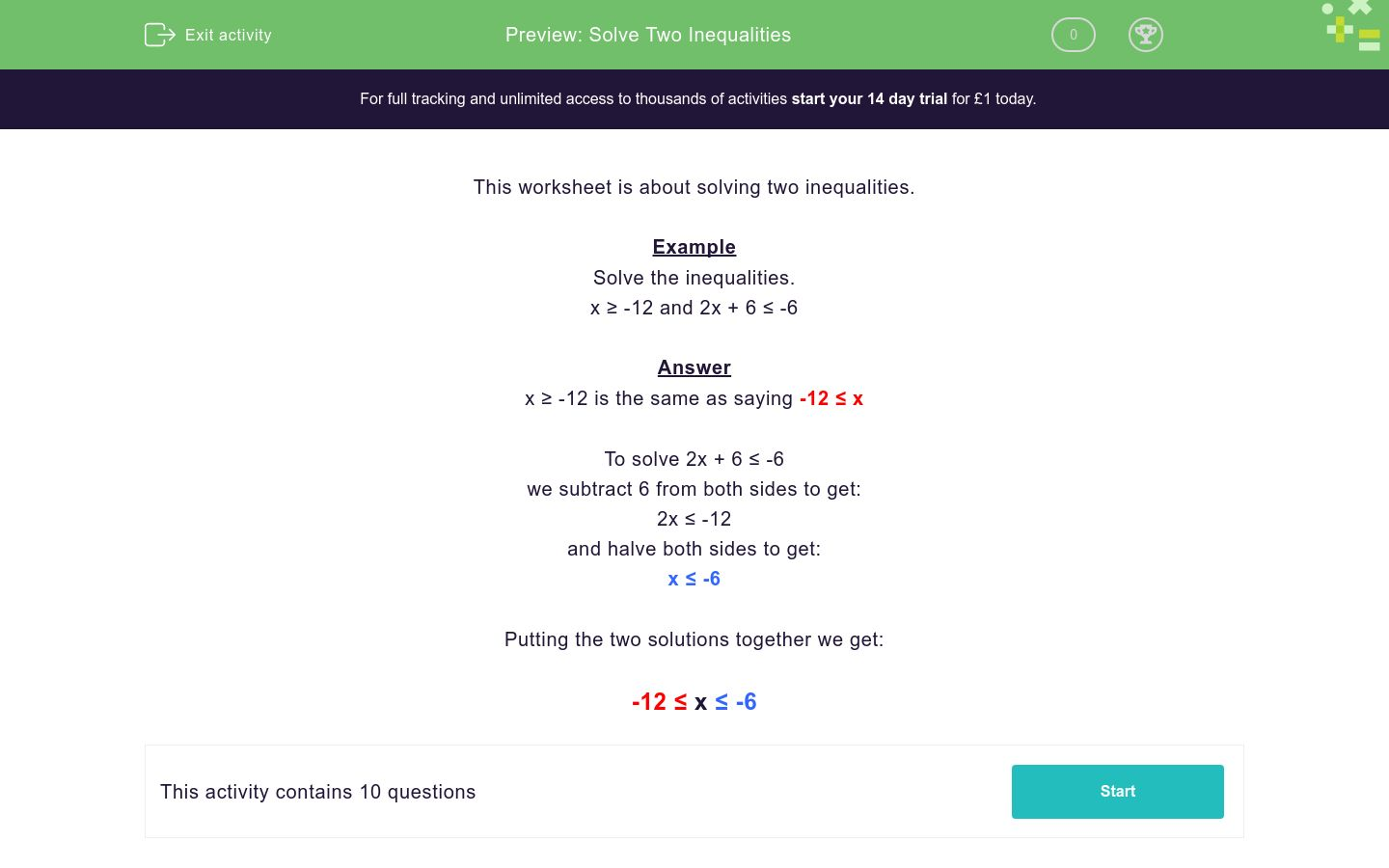# Solve Two Inequalities

In this worksheet, students solve inequalities.Key stage:  KS 3

Curriculum topic:   Algebra

Curriculum subtopic:   Understand Expressions, Equations, Inequalities, Terms and Factors

Difficulty level:### QUESTION 1 of 10

This worksheet is about solving two inequalities.

Example

Solve the inequalities.

x ≥ -12 and 2x + 6 ≤ -6

x ≥ -12 is the same as saying -12 ≤ x

To solve 2x + 6 ≤ -6

we subtract 6 from both sides to get:

2x ≤ -12

and halve both sides to get:

x ≤ -6

Putting the two solutions together we get:

-12 ≤ x ≤ -6

Solve the inequalities.

x ≤ 8 and 4x -1 > 7

10 ≤ x < 13

2 < x ≤ 8

Solve the inequalities.

x > -7 and 4x + 13 < -3

10 ≤x < 13

-7 < x < -4

Solve the inequalities.

x < 13 and 2x -7 ≥ 13

10 ≤ x < 13

-7 < x < -4

Solve the inequalities.

x ≤ -1 and 5x + 15 ≥ -10

10 ≤ x < 13

-5 ≤ x ≤ -1

Solve the inequalities.

x ≥ 1 and 2x -4 ≤ 12

10 ≤ x < 13

1 ≤ x ≤ 8

Solve the inequalities.

x ≤ 5 and 4x + 11 > -1

-3 < x ≤ 5

1 ≤ x ≤ 8

Solve the inequalities.

x ≤ -3 and 4x + 20 ≥ -4

-6 ≤ x ≤ -3

1 ≤ x ≤ 8

Solve the inequalities.

x > -9 and 5x + 9 < -6

-6 ≤ x ≤ -3

-9 < x < -3

Solve the inequalities.

x ≥ 3 and 3x -13 < 17

3 ≤ x < 10

-9 < x < -3

Solve the inequalities.

x > -10 and 6x + 7 < -17

3 ≤ x < 10

-10 < x < -4

• Question 1

Solve the inequalities.

x ≤ 8 and 4x -1 > 7

2 < x ≤ 8
• Question 2

Solve the inequalities.

x > -7 and 4x + 13 < -3

-7 < x < -4
• Question 3

Solve the inequalities.

x < 13 and 2x -7 ≥ 13

10 ≤ x < 13
• Question 4

Solve the inequalities.

x ≤ -1 and 5x + 15 ≥ -10

-5 ≤ x ≤ -1
• Question 5

Solve the inequalities.

x ≥ 1 and 2x -4 ≤ 12

1 ≤ x ≤ 8
• Question 6

Solve the inequalities.

x ≤ 5 and 4x + 11 > -1

-3 < x ≤ 5
• Question 7

Solve the inequalities.

x ≤ -3 and 4x + 20 ≥ -4

-6 ≤ x ≤ -3
• Question 8

Solve the inequalities.

x > -9 and 5x + 9 < -6

-9 < x < -3
• Question 9

Solve the inequalities.

x ≥ 3 and 3x -13 < 17

3 ≤ x < 10
• Question 10

Solve the inequalities.

x > -10 and 6x + 7 < -17

-10 < x < -4
---- OR ----

Sign up for a £1 trial so you can track and measure your child's progress on this activity.

### What is EdPlace?

We're your National Curriculum aligned online education content provider helping each child succeed in English, maths and science from year 1 to GCSE. With an EdPlace account you’ll be able to track and measure progress, helping each child achieve their best. We build confidence and attainment by personalising each child’s learning at a level that suits them.

Get started